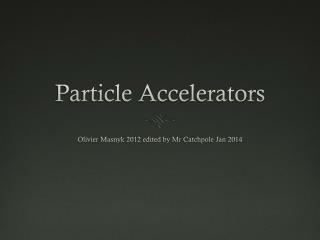DownloadDownload PresentationParticle Accelerators

# Particle Accelerators

Download Presentation## Particle Accelerators

- - - - - - - - - - - - - - - - - - - - - - - - - - - E N D - - - - - - - - - - - - - - - - - - - - - - - - - - -
##### Presentation Transcript

1. Particle Accelerators Olivier Masnyk 2012 edited by Mr Catchpole Jan 2014

2. Types of Particle Accelerators Linear (linac) Cyclotron Synchrotron

3. Linear Particle Accelerator This is a particle accelerator which accelerates a particle along a straight path with alternating current. What happens is the electron in the tube is accelerated by the pulsing of electric fields as it travels through cylindrical tubes. Each pulse is from an AC source. Thus causing a positive or negative charge. Each charge either attracts the electron or repels it. So as the electron is traveling through, it is first attracted by an opposite charge. Then as it gets to the source, the electric field changes charge to repel the electron onto the next tube.

4. Cyclotron

5. Cyclotron This method of acceleration uses increasingly large rings to send the beam through, this way the accelerator will take up less space. 2 hollow electrodes called ‘D’s Placed in uniform magnetic field Particle begins in the centre A source of alternating potential is established in the two ‘D’s Radius is larger each time due to increase in speed Potential difference is always that the ‘D’ the particle is accelerating to has an attractive potential e.g negative particle accelerates towards a positive potential difference.

6. Cyclotron adv. and disadv. Advantages are the small compact size, and therefore a relatively low cost, can be used for nuclear physics research as well as biomedical studies Disadvantages are the limit to energy they can reach due to limitations on the size of the magnets and they can only be used for fixed target experiments.

7. The Force on a Particle F=qvB As the particle is accelerated the radius increases, the particle moves on a spiral. The time to complete on rev. is found using

8. Cyclotron period If the values of v is substituted in the formula we then get: This means the period of the revolution is independent of the speed. Despite the fact that the particle is accelerating, the period T is the same.

9. Synchronising This means the potential difference between the ‘D’s changes every half period. Therefore the period of the alternating voltage source is the same as the period of the revolutions of the particle. i.e is called the cyclotron period and its inverse, is called the cyclotron frequency.

10. As the particle accelerates its radius increases At some point additional magnetic fields are placed at the edge of the magnetic field region. This will pull the charged particle out and direct it at a target. Just as it exits, the particle is travelling at it is moving at its max speed. It is also moving on the Cyclotron’s Edge.

11. KE is known Thus from we can find: So the maximum kinetic energy the particle can have is:

12. Synchrotron (CERN) • Large electro-magnets are placed along the beam to deflect the charged particles into a circular path. • In between the gaps, electrical fields are established. A bean of charged particles moves along a circular path of a fixed diameter. The ring is a thin evacuated tube.

13. Synchrotron The particles then accelerate between AB. This takes place at every gap. The potential difference have to be timed carefully. The period of the electric fields have to be synchronous with the beam, hence the name for the accelerator.

14. Energy The particles quickly reach (almost) the speed of light. At this speed the rest energy mc2is negligible compared to the kinetic energy, so the relation between the total energy and the momentum is E=pc

15. Advs. and Disadvs. Advantages of using a synchrotron are abilities like being able to accelerate particles to very high energies, and since they use colliding beams the provide very high available energies for the production of new particles. The use of storage rings means that unlike the others, the collisions can be controlled. Disadvantages are the high proportion of energy lost due to synchrotron radiation and the low probability of collisions.

16. Bremsstrahlung Known as braking radiation or synchrotron radiation Electrically charged particles, when accelerated, radiate electromagnetic waves, and so energy. Means all energy that goes into an acceleration cannot all be used, and some is wasted.

17. Production of Particles in Accelerators A proton-proton high energy collision should result in heavier particles being created: http://galileo.phys.virginia.edu/classes/252/particle_creation.html,

18. Particle energy Mass and energy are interchangeable so the total energy of a particle can be expressed as: In other words its rest mass/energy plus the energy given to it to making it accelerate (usually in eV) As the particle gets faster and v is the same order of magnitude as c its mass increases significantly so you cannot work out its velocity from this without using relativistic equations.

19. Available energy The available energy for a collision of moving particles into a stationary target is expressed (by the IB) as: M is the rest mass of the target particle E is the energy (total) of the moving particle m is the mass of the moving particle

20. Available energy • A proton is accelerated inside a large cyclotron and given a kinetic energy of 150 MeV. • What is the total energy of the proton? • What is the energy available if such a proton collides with a stationary neutron? • Is there enough energy to create a neutral pion (135MeV) in addition to the proton and the neutron?